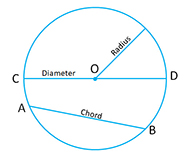It is the set of all points in a plane that are at a given distance from a given point, the center; equivalently it is the curve traced out by a point that moves so that its distance from a given point is constant. The distance between any of the points and the center is called the radius.

Perimeter of the circle is called its circumference.

Circumference of a circle$= 2 \pi r = \pi d$

Area of a circle$= \pi r^2$

In any circle, there are three types of points (refer to the diagram):

1. Exterior Points: These are points outside the circle. The distance of these points from the center is greater than the length of the radius of the circle.
2. Interior Points: These are points inside the circle.The distance of these points from the center is less than the length of the radius of the circle.
3. Points on the circumference: These are the points on the circumference. In this case, the distance of the point from the center of the circle is equal to the length of the radius of the circle.

Concentric circles: Two or more circles are called concentric if they have the same center but different radii.

Equal circles: The circles are said to be equal or congruent if they have the same radii.

Circumscribed circle: A circle that passes through all the vertices of a polygon is called a circumscribed circle. The center of this circle is called circumcenter and the polygon is called inscribed polygon.

Inscribed circle: A circle that touches all the sides of the polygon is called inscribed circle of the polygon. The center of this circle is called incenter and the polygon is called the circumscribed polygon.

Theorem 4: A straight line drawn from the center of the circle to bisect a chord, which is not the diameter, is perpendicular to the chord.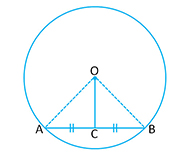Given:$AC = CB, O$ is the center of the circle

To Prove:$OC \perp AB$

Proof: Consider$\triangle OAC \ and \ \triangle OBC$$OA = OB$ (radius of the same circle)$OC$ is common$AC = BC$ (given)

Therefore$\triangle OAC \cong \triangle OBC$ (S.S.S postulate)

Therefore$\angle OCA = \angle OCB$ (corresponding angles in congruent triangles are equal)

Hence$\angle OCA = \angle OCB = 90^o$$\Rightarrow OC \perp AB$

Hence Proved.

Theorem 5: A perpendicular to a chord, from the center of the circle, bisects the chord.

Given:$OP \perp AB, \ and \ O$ is the center of the circle.To Prove:$AP = PB$

Proof: Consider$\triangle AOP \ and \ \triangle BOP$$\angle OPA = \angle OPB$ (given)$OP$ is common$OA = OB$ (radius of the circle)

Therefore$\triangle AOP \cong \triangle BOP$ (R.H.S postulate)

Therefore$AP = PB$ (congruent parts of the triangles are equal)

Therefore$OP$ bisects$AP$ and$AP=PB$

Hence Proved.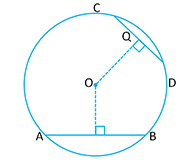Note: Greater the size of the chord, closer it is to the center of the circle and smaller the size of the chord, farther away it is from the center of the circle. In the adjoining figure,$AB > CD \Rightarrow OP < OQ$

Theorem 6: There is only one circle, and only one, which passes through three given points which are not in a straight line.

Given: Three points$A, B \ and \ C$ which are not in a straight line.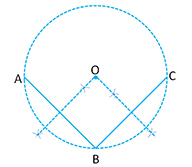To Prove: Only one circle can be drawn through$A, B \ and \ C$.

Construction: Join$AB \ and \ BC$. Draw perpendicular bisector of$AB \ and \ BC$. The perpendicular bisectors meet at$O$.

Proof:$O$ lies on the perpendicular bisector of$AB \Rightarrow OA = OB$

Also since,$O$ likes on the perpendicular bisector of$BC \Rightarrow OB=OC$

Therefore$OA=OB=OC$

Which means$O$ is equidistant from$A, B \ and \ C$.

So if you were to draw a circle with$O$as the center and$OA$ as the radius, the circle will pass through$B \ and \ C$.

The perpendicular bisector of$AB \ and \ BC$ intersect each other only at point$O$.$\Rightarrow O$ is the only point which is equidistant from$A, B \ and \ C$.

Hence, one and only one circle can be drawn through$A, B \ and \ C$ which are not in a straight line.

Hence Proved.

Note:

• The perpendicular bisector of any chord of a circle will always pass through the center of this circle.
• Perpendicular bisector of any two chords of a circle always intersect at the center of the circle.

Theorem 7: Equal chords of a circle are equidistant from the center.

Given: Chord$AB =$ Chord$CD$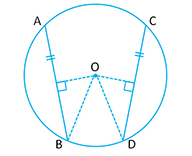To Prove: Chords$AB$ and Chords$CD$ are equidistant from the center of the circle. i.e.$OP \perp AB \ and \ OQ \perp CD$ Prove$OP = OQ$

Proof: Join$OB \ and \ OD$$BP= \frac{1}{2} AB$ ( Perpendicular from the center will always bisect the chord)

Similarly,$DQ = \frac{1}{2} CD$

But$AB = CD$ (given)

Therefore$BP =DQ$

Now consider$\triangle OPB \cong \triangle OQD$$BP=DQ$ (proved above)$OB=OD$ (radius of the circle)$\angle OPB = \angle OQD = 90^o \ (OP \perp AB \ and \ OQ \perp CD)$

Therefore$\triangle OPB \cong \triangle OQD$ (R.H.S postulate)$\Rightarrow OP=OQ$

Hence Proved.

Theorem 8 (Converse of Theorem 7) : Chords of circle which are equidistant from the center of the circle are equal in length.Given: Chords$AB \ and \ CD$ are equidistant. This means that$OP=OQ$ and$OP \perp AB \ and \ OQ \perp CD$

To Prove:$AB = CD$

Proof: Consider$\triangle OPB \ and \ \triangle OQD$$OP=OQ$ (Given)$OB=OD$ (radius of the same circle)$\angle OPB = \angle OQD= 90^o$ ($OP \perp AB \ and \ OQ \perp CD$)

Therefore$\triangle OPB \cong \triangle OQD$ (R.H.S postulate)

Therefore$PB = QD$ (Corresponding sides of congruent triangles are equal).

We know that perpendicular from the center of the circle will bisect the chord.

Therefore$\frac{1}{2} AB = \frac{1}{2} CD$$\Rightarrow \ Chord \ AB = \ Chord \ CD$.

Hence proved.Note: If parallel chords are on the same side of the circle, then the line through the midpoints of the chord will pass through the center.Note: If two equal chords$AB \ and \ CD$ intersect inside the triangle at point$P$, then we have i)$PA=PC$ and ii)$PA=PD$ i.e the corresponding segments of the chords are equal.